# ML | Boston Housing Kaggle Challenge with Linear Regression

Boston Housing Data: This dataset was taken from the StatLib library and is maintained by Carnegie Mellon University. This dataset concerns the housing prices in housing city of Boston. The dataset provided has 506 instances with 13 features.

The Description of dataset is taken from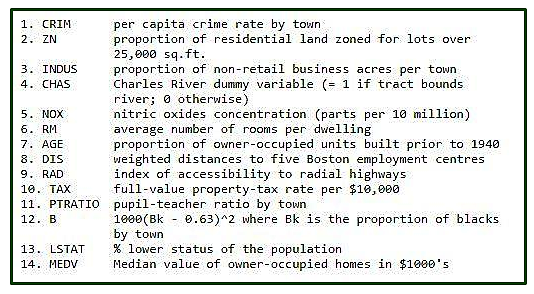Let’s make the Linear Regression Model, predicting housing prices

Inputing Libraries and dataset.

 `# Importing Libraries ` `import` `numpy as np ` `import` `pandas as pd ` `import` `matplotlib.pyplot as plt ` `  `  `# Importing Data ` `from` `sklearn.datasets ``import` `load_boston ` `boston ``=` `load_boston() `

Shape of input Boston data and getting feature_names

 `boston.data.shape `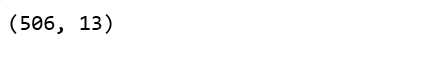`boston.feature_names `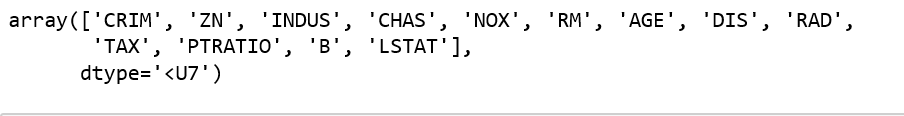Converting data from nd-array to dataframe and adding feature names to the data

 `data ``=` `pd.DataFrame(boston.data) ` `data.columns ``=` `boston.feature_names ` ` `  `data.head(``10``) `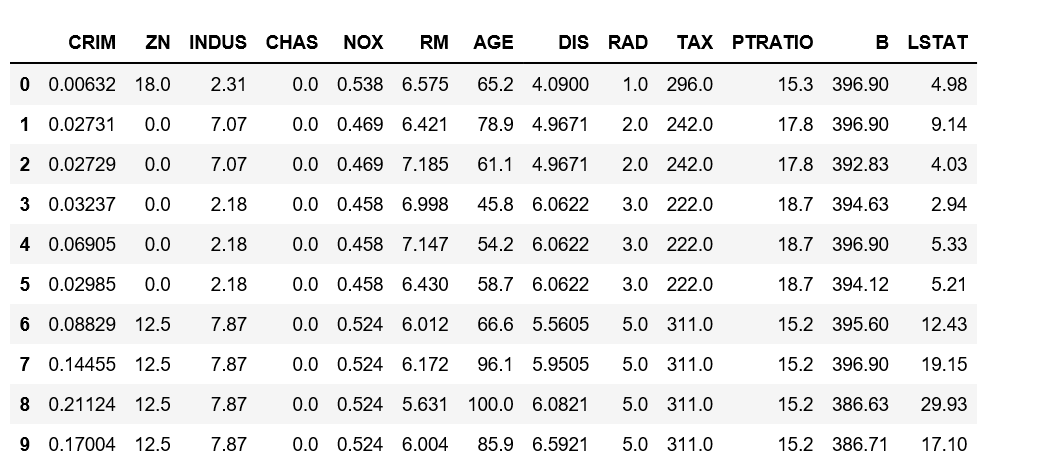Adding ‘Price’ column to the dataset

 `# Adding 'Price' (target) column to the data  ` `boston.target.shape `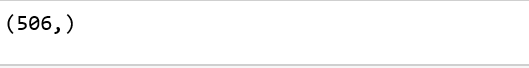`data[``'Price'``] ``=` `boston.target ` `data.head() `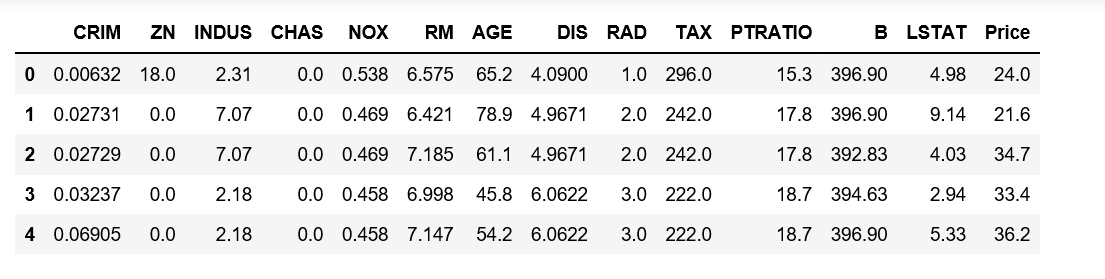Description of Boston dataset

 `data.describe() `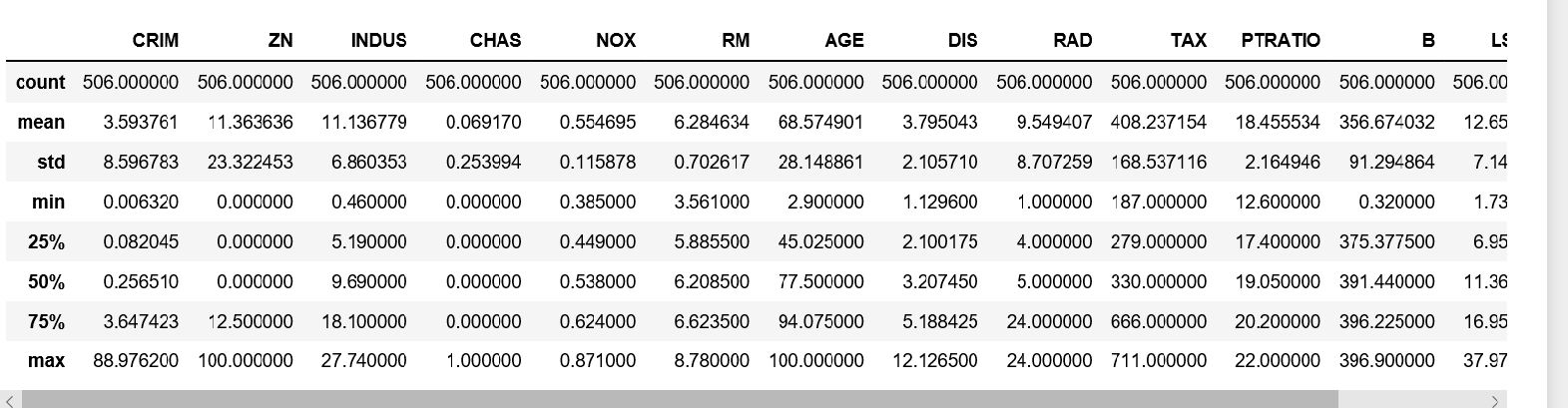Info of Boston Dataset

 `data.info() `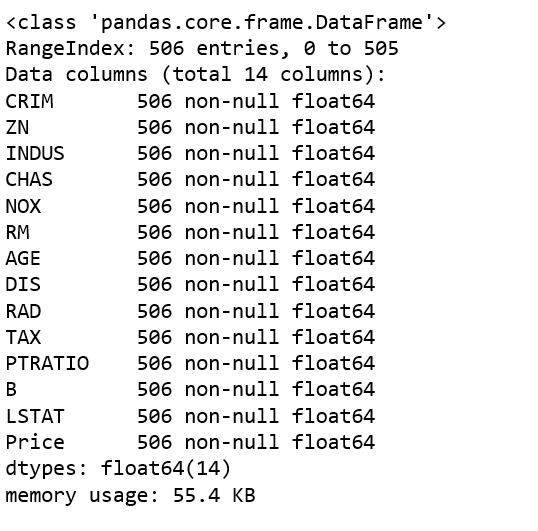Getting input and output data and further splitting data to training and testing dataset.

 `# Input Data ` `x ``=` `boston.data ` `  `  `# Output Data ` `y ``=` `boston.target ` `  `  `  `  `# splitting data to training and testing dataset.  ` `from` `sklearn.cross_validation ``import` `train_test_split ` `xtrain, xtest, ytrain, ytest ``=` `train_test_split(x, y, test_size ``=``0.2``, ` `                                                    ``random_state ``=` `0``) ` `  `  `print``(``"xtrain shape : "``, xtrain.shape) ` `print``(``"xtest shape  : "``, xtest.shape) ` `print``(``"ytrain shape : "``, ytrain.shape) ` `print``(``"ytest shape  : "``, ytest.shape) `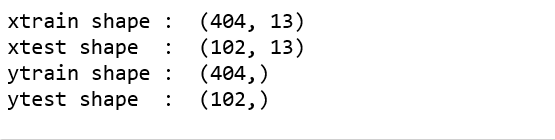Applying Linear Regression Model to the dataset and predicting the prices.

 `# Fitting Multi Linear regression model to training model ` `from` `sklearn.linear_model ``import` `LinearRegression ` `regressor ``=` `LinearRegression() ` `regressor.fit(xtrain, ytrain) ` `  `  `# predicting the test set results ` `y_pred ``=` `regressor.predict(xtest) `

Plotting Scatter graph to show the prediction results – ‘ytrue’ value vs ‘y_pred’ value

 `# Plotting Scatter graph to show the prediction  ` `# results - 'ytrue' value vs 'y_pred' value ` `plt.scatter(ytest, y_pred, c ``=` `'green'``) ` `plt.xlabel(``"Price: in \$1000's"``) ` `plt.ylabel(``"Predicted value"``) ` `plt.title(``"True value vs predicted value : Linear Regression"``) ` `plt.show() `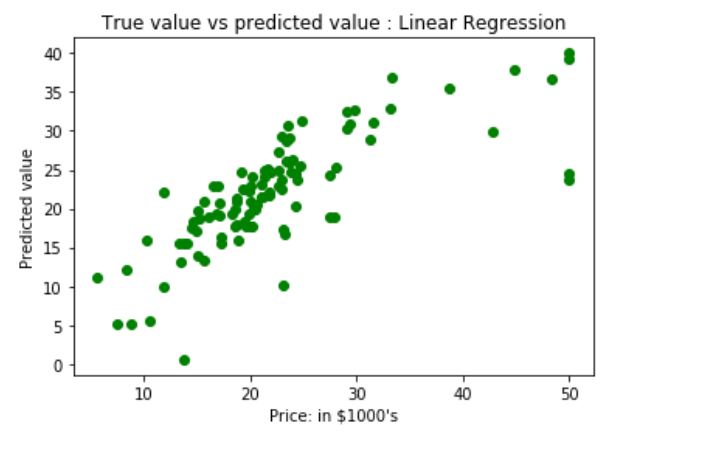Results of Linear Regression i.e. Mean Squred Error.

 `# Results of Linear Regression. ` `from` `sklearn.metrics ``import` `mean_squared_error ` `mse ``=` `mean_squared_error(ytest, y_pred) ` `print``(``"Mean Square Error : "``, mse) `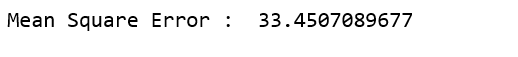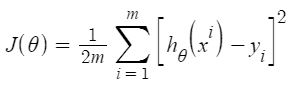As per the result our model is only 66.55% accurate. So, the prepared model is not very good for predicting the housing prices. One can improve the prediction results using many other possible machine learning algorithms and techniques.

My Personal Notes arrow_drop_upIf you like GeeksforGeeks and would like to contribute, you can also write an article using contribute.geeksforgeeks.org or mail your article to contribute@geeksforgeeks.org. See your article appearing on the GeeksforGeeks main page and help other Geeks.

Please Improve this article if you find anything incorrect by clicking on the "Improve Article" button below.